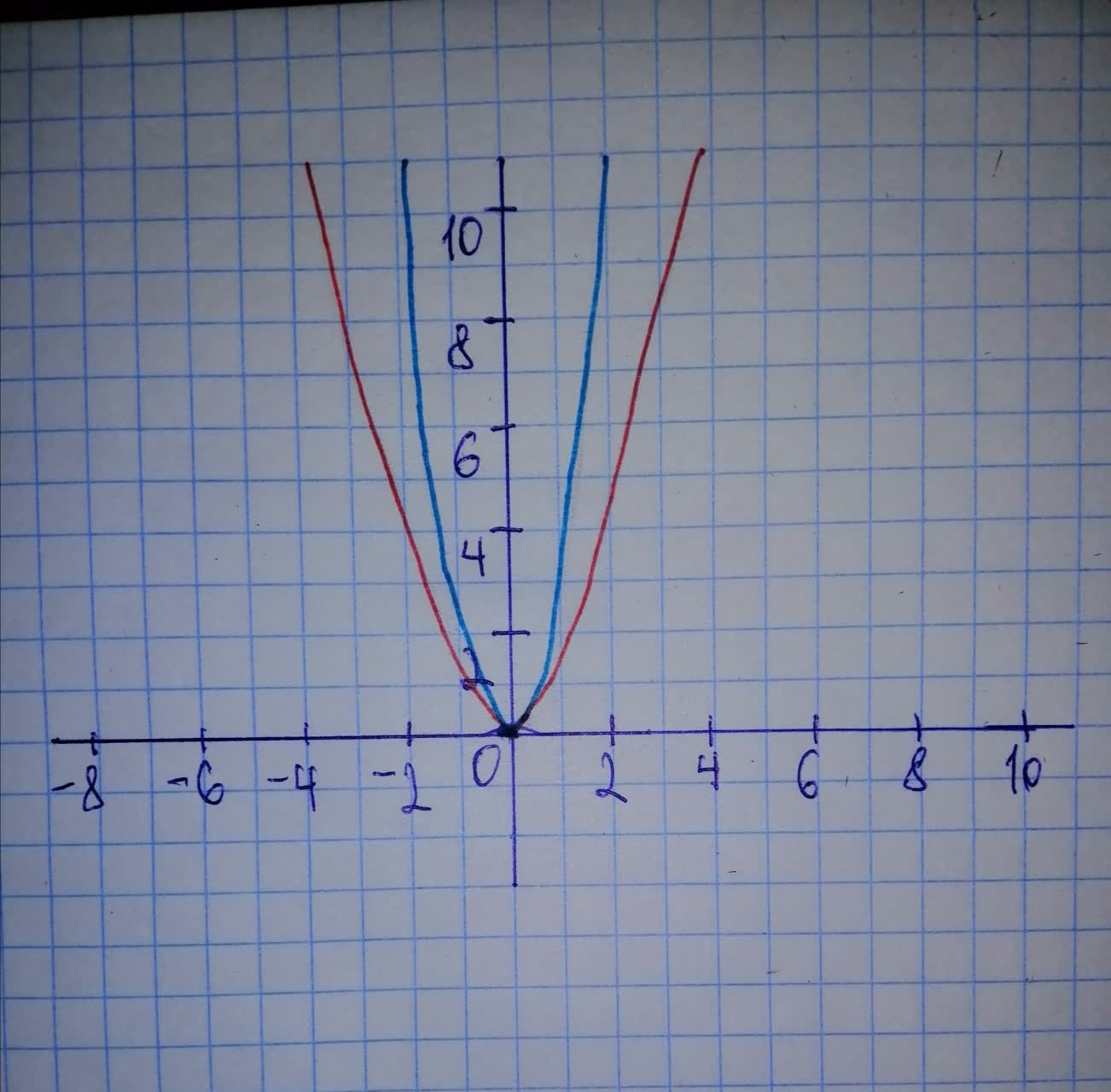Question# Sketch a graph of the function. Use transformations of functions whenever possible. f(x)=3x^{2}

Transformations of functions
ANSWEREDSketch a graph of the function. Use transformations of functions whenever possible. $$\displaystyle{f{{\left({x}\right)}}}={3}{x}^{{{2}}}$$First graph function $$\displaystyle{y}={x}^{{{2}}}$$ Then, to obtain graph of function $$\displaystyle{f{{\left({x}\right)}}}={3}{x}^{{{2}}}$$, do following transformations: - stretc vertically by a factor of 3 On graph: Red - $$\displaystyle{y}={x}^{{{2}}}$$ Blue - $$\displaystyle{f{{\left({x}\right)}}}={3}{x}^{{{2}}}$$